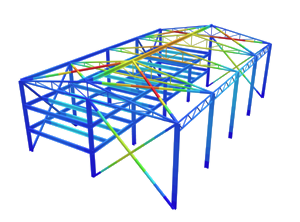Documentazione SkyCiv

La tua guida al software SkyCiv - tutorial, guide pratiche e articoli tecnici

1. Casa
2. Esercitazioni
3. Beam Tutorials
4. Che cos'è il momento flettente?

# Che cos'è il momento flettente?

## Che cos'è il momento flettente?

In this tutorial, we will simply answer the question: What is a bending moment? A bending moment is a force normally measured in a force x length (per esempio. kNm). Bending moments occur when a force is applied at a given distance away from a point of reference; causing a curvatura effect. In the most simple terms, a bending moment is basically a force that causes something to bend. If the object is not well-restrained the bending force will cause the object to rotate about a certain point. It might also be worth noting you can experiment and try our free calculator to calculate shear and bending moment diagrams of a beam.

This is often a difficult idea to comprehend, therefore consider an example of a plastic ruler overhanging a desk. If one end of the ruler is lying on the desk and is being held down, and then a force is applied to the other end of the ruler, it will cause the ruler to bend. The ruler will experience the greatest bending moment at the end where the force is applied.To calculate the bending moment about a reference point, we take the magnitude of the force and multiply it by the distance of the force from the point. Often this is calculated across the span of a member, here you can learn how to draw bending moment diagrams. Note that the force must be perpendicular to the line between the point where the force is applied and the reference point. Though that sounds confusing, it can be simplified in our example:In the above example, the bending moment at point A is simply the distance multiplied by the force. Perciò, the Bending Moment at Point A = 0.2(10) = 2 nm.

It is important to note that to use the above formula, the force (in this case a 10 N downward force) must NOT pass through the point. This is obvious as if we applied the same formula (distance x force) the distance would equal zero and therefore there would be no bending moment force.

Questo articolo ti è stato utile?

# Come possiamo aiutare?

### Prova SkyCiv oggiPotente, software di analisi strutturale e progettazione basato sul web